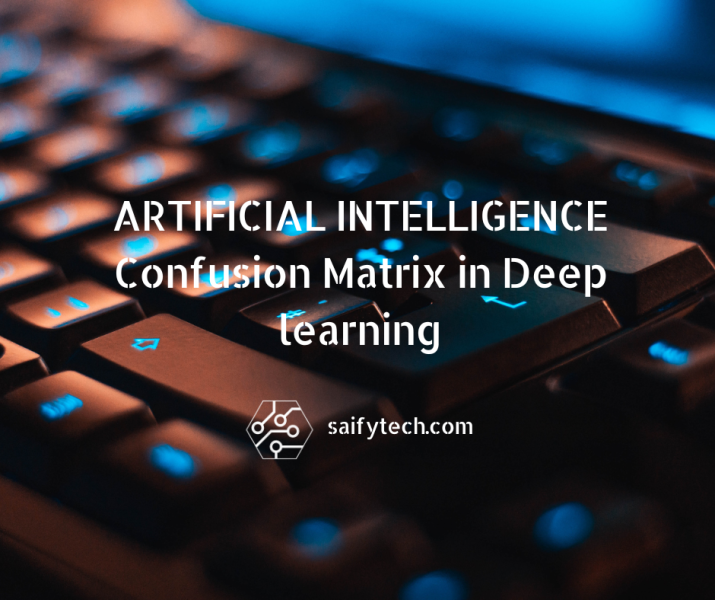# Confusion Matrix in Deep learning

Confusion Matrix is mainly used machine language and deep learning field to evaluate the perfomance of model ,by showing all its predicted value in a table.

It is mainly used to evaluate classification model(classifier) by calculating precision,recall and f1 score using confusion matrix.
As name suggest it create confusion in terminalogy which is used in table.when i was trying to understand confusion matrix by just 2 items it create too much confusion
for me so i used 3 items and to my surprise 3 items make it too easy understand.

we will cover following question in this post

1. what is confusion matrix ?
2. how to create confusion matrix with binary classification example ?
3. how to create confusion matrix with more than 2 classification example ?.
4. how to calculate precision,recall and f1 score through confusion matrix ?

## 1) what is confusion matrix ?

confusion matrix is reprentation of the classifier model's predicted value and real value in a table like structure where all rows represent predicted value and all colums represents real values , all value presented in the table is the number of Input provided to the model for classification

Table shown above is the confusion matrix used to predict dog vs cat

## 2) how to create confusion matrix with binary classification example ?

In the dog vs cat example we have designed and trained model to predict two values which is either dog or cat . To create confusion matrix we need to take one item at a time .In the our case we are taking first dog then we will create four values that is TP,TN,FP,FN.
there are four terminology used in confusion matrix ehich is True positive(TP), True Negative(TN),False positive(FP),False Negative(TN),

1. True positive(TP):- All those value predicted by model is matching with real values means model predict it correctly.Like in above case it is 28
In which dog is predicted as dog.
2.  True Negative(TN):-All those value predicted by model is not dog and its real value is also not dog which is cat like in the above case it is 22
3.  False positive(FP):-All those value predicted by model is not dog but the real value is dog which in the case is 10
4.  False Negative(TN):-All those value predicted by model is dog but in reality it is cat which in the case is 4.

As for dog all four value are
TP=28
TN=22
FP=4
FN=10
TOTAL=64

As for cat will be
TP=28
TN=22
FP=4
FN=10
TOTAL=64

## 3)how to create confusion matrix with more than 2 classification example ?

In this example we have designed and trained model for three items classifier which is dog,cat and buffalo. We now have 100 input items and the models prediction for 100 items is given below in confusion matrix as.

a)  As we have seen in the above image. if we consider dog as our entity or classifier than
TP=30, TN=28+5+3+25, FP=2+3, FN=2+2.

b) now if we consider cat as a classifier than we will get
TP=28, TN=25+3+30+2, FP=2+3, FN=2+5.

c) now if we consider buffalo as a classifier than we will get
TP=25, TN=30+2+2+28, FP=2+3, FN=3+5.

## 4) how to calculate precision,recall and f1 score through confusion matrix ?

precision= TP/TP+FP
precision shows how precise our model is on our input means.

Recall= TP/TP+FP
recall shows how many times model is correct in all real values.

f1 score =2*precision*Recall
----------------
precision+Recall

f1 gives combination of precision and recall . it gives more weightage to prescision.

Lets find preicision and recall for both models which we have mentioned above .Lets calculate precision for dog in dog/cat prediction model.

precision(dog)= 28/28+4 = 28/32=0.8
Recall(dog)= 28/28+10 = 28/38=0.73

Lets find preicision for cat in dog/cat prediction model.

precision(cat)= 22/22+10 = 22/32=0.68
Recall(cat)= 22/22+4 = 22/26=0.84

Models total precision and recall will be means as it

precision(cat)= 0.8+0.68/2 = 0.74
Recall(cat)= 0.73+0.84/2= 0.78

Kindly Comments us if you are facing any problems in understanding confusion matrix ,We will be happy to help you.